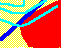APPENDIX A: EXPECTED UTILITY THEOREM

Expected utility theory posits a set of rational choice axioms. If these assumptions are satisfied, then the expected utility theorem shows the existence of a utility function with the elegant property that the preferences over risky outcomes can be represented by the expected utility of these outcomes.

A binary investment opportunity, or a binary lottery, consists of three numbers: two outcomes and a probability of the first outcome. We denote this as L = {p,x,y}, where p is the probability of outcome x (so (1-p) is the probability of y).

We can also combine lotteries. This is called a compound lottery. Thus, the compound lottery L = {p,L1,L2} lets you win the lottery L1 with probability p, and L2 with probability (1-p). When we combine lotteries L1 and L2 to create L, we denote this asAxioms

1. Complete ordering axiom. For any pair of lotteries L1 and L2, one of the following is true: L1 is strictly preferred to L2 , L2 is strictly preferred to L1, or the investor is indifferent between the two lotteries.

2. Continuity axiom. For three lotteries, assume that L1 is strictly preferred to L2, and L2 is strictly preferred to L3. Then there exists some probability p so that the investor is indifferent between L2 for sure and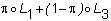.

3. Independence axiom. Suppose the investor is indifferent between lotteries L1 and L2. Let L3 be another lottery. Then, the investor is indifferent betweenand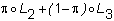Similarly, if L1 is strictly preferred to L2, then the first combination is strictly preferred to the second.

4. Unequal probability axiom. Assume that L1 is strictly preferred to L2. If Li = {p , L1, L2} and Lk = {r, L1, L2 } then Li is strictly preferred to Lk if and only if p > r.

The set of possible risky investment opportunities is assumed to be finite. Denote the most preferred (best) as LB and the least preferred (worst) as LW.

Expected Utility Theorem

If these axioms are satisfied, then there exists a utility function, U, such that for any two lotteries L1 and L2, L1 is preferred to L2 if and only if the expected utility from L1 is greater than that from L2,

Proof:

Set U(LB) = 1 and U(Lw) = 0. Now, consider a lottery L1. According to the continuity axiom, there exists a probability, say p1, such that the investor is indifferent between L1 and the compound lottery opportunity (p1,LB,Lw).

Set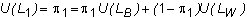Similarly, for any other lottery L2, we set U(L2) = p2, where the investor is indifferent between L2 and (p2 ,LB , Lw ).

We want to make sure that the way we are assigning utility numbers preserves the investor's preferences. From Axiom 1, the investor is either indifferent between L1 and L2 or strictly prefers L1 or strictly prefers L2. By Axiom 4, this will be the case only if respectively p1 = p2 , p1 > p2 , or p1 < p2, and so U preserves the preferences.

Finally, we need to show that we can compare any two compound lotteries simply by taking expected utilities. This requires us to show that if L = (p, L1, L2) is a compound lottery, then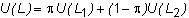To show this, we need the independence axiom. First, substitute (p1, LB, Lw) for L1, and (p2, LB, Lw ) for L2. Then, the investor is indifferent between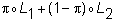and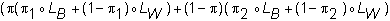which equals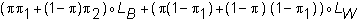which is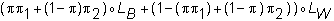or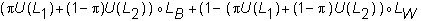By construction U(LB )=1 and U(Lw)=0. Therefore, this equals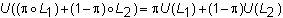which establishes the expected utility theorem.

previous topic

next topic